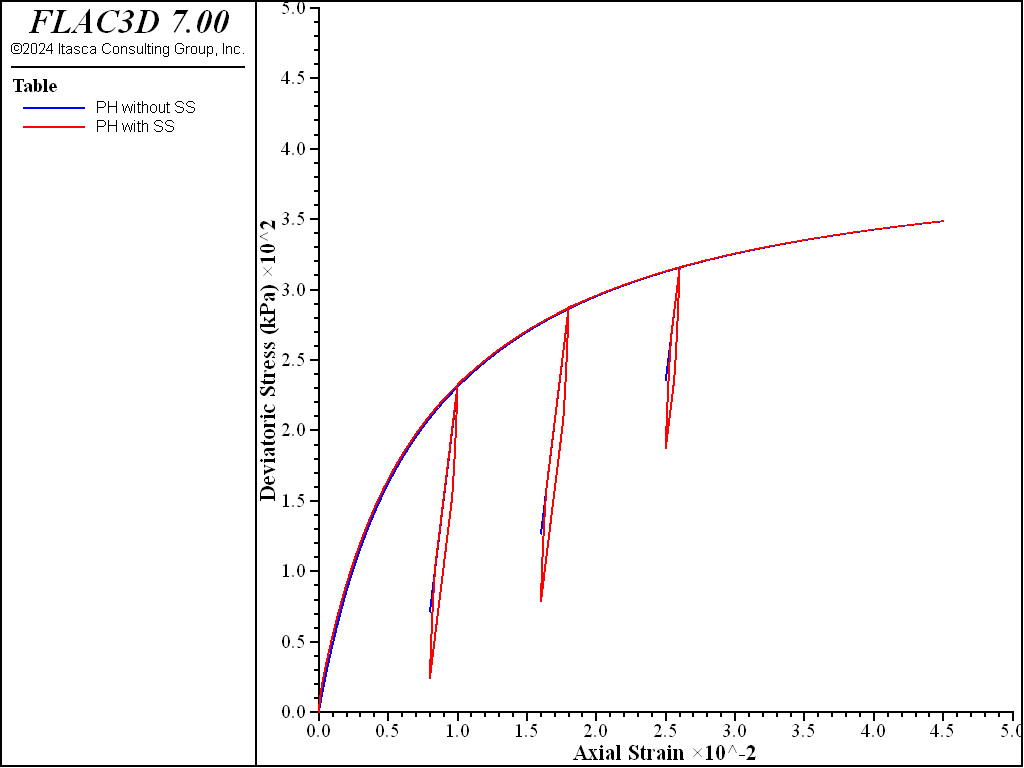FLAC3D Theory and Background • Constitutive Models

# Comparison of Plastic-Hardening Model without and with Small-Strain Stiffness

Note

To view this project in FLAC3D, use the menu command Help > Examples…. Choose “ConstitutiveModels/ ComparisonSmallStrainPlasticHardening” and select “ComparisonSmallStrainPlasticHardening.prj” to load. The project’s main data files are shown at the end of this example.

This example compares the behavior of the Plastic-Hardening (PH) model without and with small-strain stiffness during triaxial compression. Both models comprise a one-zone triaxial compression test with a constant cell pressure of 100 kPa. Case 1 is the standard PH model without small-strain stiffness, and Case 2 is the PH model with small-strain stiffness. The parameters are listed in Table 1.

 Basic Parameters: $$\phi$$ (degrees) 30 $$c$$ (kPa) 0 $$\psi$$ (degrees) 10 $$E^{ref}_{50}$$ (kPa) 3e4 $$E^{ref}_{ur}$$ (kPa) 8e4 $$\nu$$ 0.25 $$m$$ 0.55 $$p^{ref}$$ (kPa) 100 Small-Strain Parameters: $$E^{ref}_{0}$$ (kPa) 3e5 $$\gamma_{70}$$ (default) 2e-4

Figure 2 shows the elastic shear moduli for both cases. In Case 1, the elastic shear modulus keep constant with a value of $$G_{ur}$$, but in Case 2 the elastic shear modulus decreases from the initial value of $$G_{0}$$ to the value of $$G_{ur}$$, and then keep the value of value of $$G_{ur}$$ as expected.Figure 1: Comparison of deviatoric stresses without and with small-strain stiffness.Figure 2: Comparison of elastic moduli without and with small-strain stiffness.

Data Files

TriaxialCompressionSmallStrainPlasticHardening.dat

model new
model large-strain off
zone create brick size 1 1 1
zone cmodel assign plastic-hardening
zone property stiffness-50-reference=3.0e4 stiffness-ur-reference=8.0e4
zone property pressure-reference=100.0 exponent=0.55 poisson=0.25
zone property coefficient-normally-consolidation=0.40
zone property friction=40.0 dilation=10.0 cohesion=0.0
zone property stress-1-effective=-100.0 stress-2-effective=-100.0 ...
stress-3-effective=-100.0
zone property flag-smallstrain=true stiffness-0-reference=30.0e4 ...
strain-70=2e-4
;
zone gridpoint fix velocity-z
zone face apply stress-xx=-100.0 range union position-x 0 position-x 1
zone face apply stress-yy=-100.0 range union position-y 0 position-y 1
zone initialize stress xx -100.0 yy -100.0 zz -100.0
;
[global gp_ = gp.find(8)]
fish define hhhq_
global hhhq_ = zone.stress.xx(zp_) - zone.stress.zz(zp_)
global hhha_ = -gp.disp.z(gp_)
global G_ = zone.prop(zp_,'shear')
global strain_ = zone.strain.shear.inc(zp_)
end
history interval 5
fish history hhhq_
fish history hhha_
fish history G_
fish history strain_
;
zone gridpoint initialize velocity-z -4e-7 range position-z 1
model step 25000
zone gridpoint initialize velocity-z  4e-7 range position-z 1
model step 5000
zone gridpoint initialize velocity-z -4e-7 range position-z 1
model step 25000
zone gridpoint initialize velocity-z  4e-7 range position-z 1
model step 5000
zone gridpoint initialize velocity-z -4e-7 range position-z 1
model step 25000
zone gridpoint initialize velocity-z  2e-7 range position-z 1
model step 5000
zone gridpoint initialize velocity-z -4e-7 range position-z 1
model step 50000
;
hist export '1' vs '2' table 'phs_qs_100'
table 'phs_qs_100' export 'phs_qs_100' truncate
hist export  '3' vs '4' table 'phs_Gs_100'
table 'phs_Gs_100' export 'phs_Gs_100' truncate
;
model save 'phs_w100'